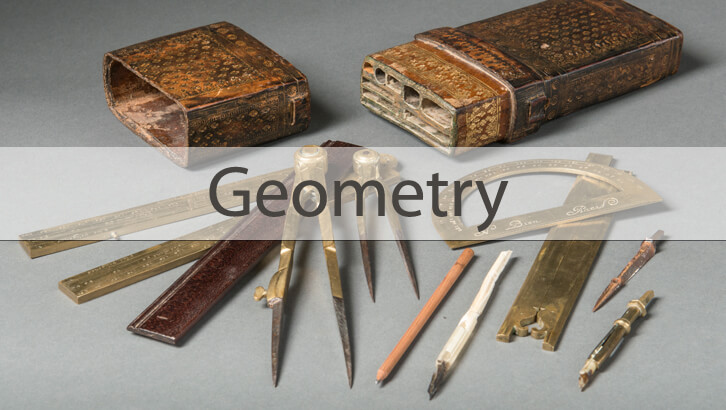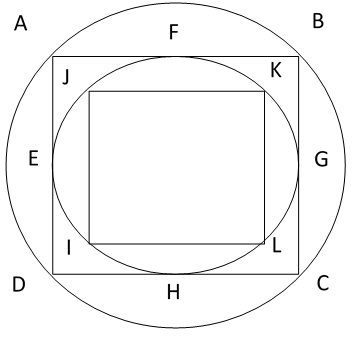# Ratio of Areas of a square in a circle and a Circle in a square

Consider the following diagram:Let side of Square ABCD be x. The diameter of the circle circumscribing it will be equal to the diagonal of the square, which is equal to$\sqrt { 2 }x$.

So, the area of the circle:$\frac { \pi { D }^{ 2 } }{ 4 } =\frac { 2\pi { x }^{ 2 } }{ 4 } =\frac { \pi { x }^{ 2 } }{ 2 }$.

Area of the square: x2.

Ratio of the area of the square ABCD to the circle circumscribing it: x2$\frac { \pi { x }^{ 2 } }{ 2 }$ = 2:$\pi$

Now, consider the circle – EFGH. The diameter of this circle will be equal to the side of the square ABCD.

So, area of this circle:$\frac { \pi { D }^{ 2 } }{ 4 } =\frac { \pi x^{ 2 } }{ 4 }$

Ratio of the square ABCD to the circle inscribed in it: x2:$\frac { \pi x^{ 2 } }{ 4 }$

= 4:$\pi$

Additionally, the side of square ABCD is the diagonal of the square IJKL, so, their areas will be in the ratio of 2:1

Ratio of areas of the circles ABCD and EFGH is also 2:1

As a general rule,

• Ratio of the area of a square to the circle circumscribing it: 2:$\pi$
• Ratio of the square to the circle inscribed in it: 4:$\pi$
• If the pattern of inscribing squares in circles and circles in squares is continued, areas of each smaller circle and smaller square will be half the area of the immediately bigger circle and square respectively.